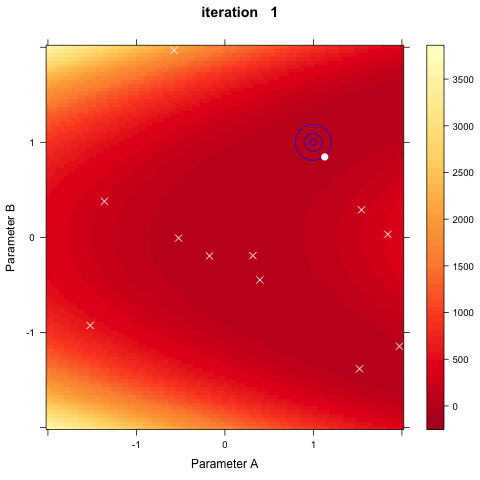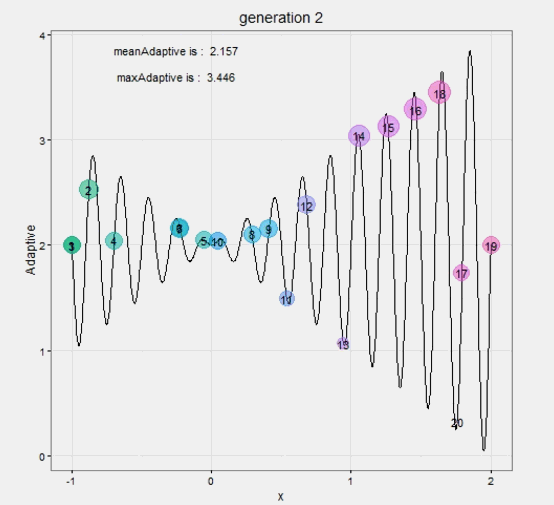“Bees don’t swarm in a mango grove for nothing. Where can you see a wisp of smoke without a fire?”

– Hla Stavhana

# PSO

PSO算法最初是为了图形化的模拟鸟群优美而不可预测的运动。而通过对动物社会行为的观察，发现在群体中对信息的社会共享提供一个演化的优势，并以此作为开发算法的基础。通过加入近邻的速度匹配、并考虑了多维搜索和根据距离的加速，形成了PSO的最初版本。之后引入了惯性权重*w*来更好的控制开发（exploitation）和探索（exploration），形成了标准版本。

PSO算法采用实数求解，不要求目标函数可微，而且模型参数的数量还行，原理有趣，易于实现，可以解决大规模、非线性、不可微和多峰值的复杂优化问题。该算法和其它全局优化算法一样可能陷入局部最优，后期收敛速度较慢，但是这个算法的思想还是很有意思的，非常值得学习。

## Principle

 1 2   vid+1 = w∙vid+c1∙rand()∙(pid-xid)+c2∙Rand()∙(pgd-xid) xid+1 = xid+vid 

1. 第一部分为微粒先前行为的惯性
2. 第二部分为“认知（cognition）”部分，表示微粒本身的思考。“认知”部分可以由Thorndike的效应法则（law of effect）所解释，即一个得到加强的随机行为在将来更有可能出现。这里的行为即“认知”，并假设获得正确的知识是得到加强的，这样的一个模型假定微粒被激励着去减小误差；
3. 第三部分为“社会（social）”部分，表示微粒间的信息共享与相互合作。“社会”部分可以由Bandura的替代强化（vicarious reinforcement）所解释。根据该理论的预期，当观察者观察到一个模型在加强某一行为时，将增加它实行该行为的几率。即微粒本身的认知将被其它微粒所模仿。

PSO算法使用如下心理学假设：在寻求一致的认知过程中，个体往往记住自身的信念，并同时考虑同事们的信念。当其察觉同事的信念较好的时候，将进行适应性地调整。这就是这个算法有趣的核心。

## Procedure

1. 初始化一群微粒（群体规模为m），包括随机的位置和速度；
2. 评价每个微粒的适应度；
3. 对每个微粒，将它的适应值和它经历过的最好位置pbest的作比较，如果较好，则将其作为当前的最好位置pbest
4. 对每个微粒，将它的适应值和全局所经历最好位置gbest的作比较，如果较好，则重新设置gbest的索引号；
5. 根据方程（1）变化微粒的速度和位置；
6. 如未达到结束条件（通常为足够好的适应值或达到一个预设最大代数Gmax），回到2）。

# Demo

## pso::psoptim()

 1  psoptim(par, fn, gr = NULL, …, lower = -1, upper = 1, control = list())
param meaning
par Vector with length defining the dimensionality of the optimization problem. Providing actual values of par are not necessary (NA is just fine). Included primarily for compatibility with optim but if values are provided within the lower and upper bounds then the first particle will be initialized to the position provided by par.
fn A function to be minimized (or maximized), with first argument the vector of parameters over which minimization is to take place. It should return a scalar result.
gr A function to return the gradient if local search is BFGS. If it is NULL, a finite-difference approximation will be used.
... Further arguments to be passed to fn and gr.
lower Lower bounds on the variables.
upper Upper bounds on the variables.
control A list of control parameters. See “Details”.
• par 定义最佳化问题的维度，一般用NA就可以了
• fn 适应值函数（最佳化的目标函数）
• gr 如果局部搜索使用的是BFGS，那么这个参数表示返回对应梯度的函数。如果该参数为NULL，表示将使用有限差分的方法
• lower upper 搜索范围
• control 其他选项的控制，选项如下：
• trace 是否显示每次迭代的过程，显示(trace=1)不显示(trace = 0)，预设为0
• fnscale 最大化(fnscale = -1, 值为-1倍)或最小化(fnscale = 1)，预设为1，如果为2，那么values = values/2
• maxit 最大迭代次数，预设maxit=1000
• maxf 停止的条件，适应值函数的最大值，预设Inf
• abstol 停止的条件，适应值函数的最小值，预设-Inf
• REPORT 显示迭代过程的步长，预设50
• s 粒子群的大小（数量），预设floor(10+2*sqrt(length(par)))
• w 粒子移动速度的权重，预设1/(2*log(2))
• cp 个体最佳解的权重，预设5+log(2)
• cg 整体最佳解的权重，预设5+log(2)
• v.max 移动速度的大小，预设v.max*d

Details

  1 2 3 4 5 6 7 8 9 10 11 12 13 14 15 16 17 18 19 20 21 22 23 24 25 26 27 28 29 30 31 32 33 34 35 36 37 38 39 40 41 42 43 44 45 46 47 48 49 50 51 52 53 54 55 56 57 58 59 60 61 62 63 64 65 66 67 68 69 70 71 72 73 74 75 76 77 78 79 80 81 82 83 84 85 86 87 88 89 90 91 92 93 94 95 96 97 98 99 100 101 102 103 104 105 106 107 108 109 110 111 112 113 114 115 116 117 118 119 120 121 122 123 124 125 126 127 128 129 130 131 132 133 134 135 136 137 138 139 140 141 142 143 144 145 146 147 148 149 150 151 152 153  library(rgl) x <- (-100:100) y <- (-100:100) z <- matrix(mapply(function(i) { mapply(function(v0) { return(sqrt(i^2 + v0^2)) }, y) }, x), nrow = 201, byrow = T ) open3d() #> wgl #> 1 surface3d(x, y, z, back = "lines", color = terrain.colors(z^0.2)) #1.初始化粒子群（包含20个粒子） vmax<-30 pbest<-NULL gbest<-NULL gbest.add<-NULL w<-1 #设置惯性权重 c1<-c2<-2 #设置加速度常数 iters<-1000#设置最大迭代次数 alpha<-0.001#设置最佳适应度值的增量阈值 xMat<-matrix(c(x=runif(20,-100,100),y=runif(20,-100,100)),byrow=F,ncol=2, dimnames=list(NULL,c("x","y"))) vMat<-matrix(c(x=runif(20,-vmax,vmax),y=runif(20,-vmax,vmax)),byrow=F,ncol=2, dimnames=list(NULL,c("x","y"))) #2.计算种群中所有粒子的适应度 adjusts<-apply(xMat,1,function(v){1/sqrt(sum(v^2)+1)}) #3.更新pbest、gbest，同时更新所有粒子的位置与速度 pbest<-xMat pbest<-cbind(pbest,adjusts) gbest<-pbest[which.max(pbest[,3]),] for(k in 1:iters) { #---更新pbest #遍历adjusts，如果对应粒子的适应度是历史中最高的，则完成替换 mapply(function(no,adj){ if(adj>pbest[no,3]) { pbest[no,]<<-c(xMat[no,],adj) #print("--更新pbest") } },1:length(adjusts),adjusts) #print("-----------pbest-----------") #print(pbest) #---更新gbest if(max(pbest[,3])>gbest){ gbest.add<-max(pbest[,3])-gbest gbest<-pbest[which.max(pbest[,3]),] print("--更新gbest") print(gbest.add) } #print("-----------gbest-------------") #print(gbest) #画出对应位置的点 plot(xMat[,1],xMat[,2],pch=20,col='blue',xlim=c(-100,100),ylim=c(-100,100)) points(gbest,gbest,pch=8,col='red') points(0,0,pch=20,cex=0.5) points(0,0,pch=21,cex=2) dev.off() #--更新所有粒子的位置与速度 old.xMat<-xMat xMat<-xMat+vMat vMat<-w*vMat+c1*runif(1,0,1)*(pbest[,1:2]-old.xMat)+c2*runif(1,0,1)* (matrix(rep(gbest[1:2],20),ncol=2,byrow=T)-old.xMat) #----如果vMat有值超过了边界值，则设定为边界值 vMat[vMat<(-vmax)]<-(-vmax) vMat[vMat>vmax]<-vmax #计算更新后种群中所有粒子的适应度 adjusts<-apply(xMat,1,function(v){1/sqrt(sum(v^2)+1)}) #检查全局适应度的增量，如果小于0.0002，则算法停止 if(!is.null(gbest.add) && gbest.add<0.0002){ print(paste("k=",k,"算法结束！")) break; } } #>  "--更新gbest" #> adjusts #> 0.02892844 #>  "--更新gbest" #> adjusts #> 0.005120944 #>  "--更新gbest" #> adjusts #> 0.02275194 #>  "--更新gbest" #> adjusts #> 0.1004123 #>  "--更新gbest" #> adjusts #> 0.03512447 #>  "--更新gbest" #> adjusts #> 0.04253347 #>  "--更新gbest" #> adjusts #> 0.2045966 #>  "--更新gbest" #> adjusts #> 0.00544671 #>  "--更新gbest" #> adjusts #> 0.1941632 #>  "--更新gbest" #> adjusts #> 0.06251679 #>  "--更新gbest" #> adjusts #> 0.1545025 #>  "--更新gbest" #> adjusts #> 0.09263582 #>  "--更新gbest" #> adjusts #> 0.006755617 #>  "--更新gbest" #> adjusts #> 9.462714e-05 #>  "k= 220 算法结束！" gbest[1:2] #> x y #> 0.06911701 0.04933046 library(pso) psoObj <- psoptim(rep(NA, 2), function(x) sqrt(x^2 + x^2), lower = c(-100, -100), upper = c(100, 100), control = list(s = 50) ) psoObj #> $par #>  1.149967e-49 -5.720875e-49 #> #>$value #>  5.835309e-49 #> #> $counts #> function iteration restarts #> 50000 1000 0 #> #>$convergence #>  2 #> #> $message #>  "Maximal number of iterations reached" Created on 2019-05-03 by the reprex package (v0.2.1) ### 2 当然也可以这样玩，在维数D较少的时候最好可视化以下方便查看：   1 2 3 4 5 6 7 8 9 10 11 12 13 14 15 16 17 18 19 20 21 22 23 24 25 26 27 28 29 30 31 32 33 34 35 36 37 38 39 40 41 42 43 44 45 46 47 48 49 50 51 52 53 54  library(pso) formula <- function(x) x*sin(10*pi * x) + 2 # curve(formula, -1, 2) psoptim(rep(NA, 1), function(x) x*sin(10*pi * x) + 2, lower = 0, upper = 2, control = list(fnscale = -1, maxit = 100), ) #>$par #>  1.850547 #> #> $value #>  -3.850274 #> #>$counts #> function iteration restarts #> 1200 100 0 #> #> $convergence #>  2 #> #>$message #>  "Maximal number of iterations reached" formula2 <- function(x) ifelse(x<1, x, 2-x) # curve(formula2, 0, 2) psoptim(rep(NA, 1), function(x) ifelse(x<1, x, 2-x), lower = 0, upper = 2, control = list(s = 1, fnscale = -1), ) #> $par #>  0.5014604 #> #>$value #>  -0.5014604 #> #> $counts #> function iteration restarts #> 1000 1000 0 #> #>$convergence #>  2 #> #> \$message #>  "Maximal number of iterations reached"

Created on 2019-05-03 by the reprex package (v0.2.1)### 3

  1 2 3 4 5 6 7 8 9 10 11 12 13 14 15 16 17 18 19 20 21 22 23 24 25 26 27 28 29 30 31 32 33 34 35 36 37 38 39 40 41 42 43 44 45 46 47 48 49 50 51 52 53 54 55 56 57 58 59 60 61 62 63 64 65 66 67 68 69 70 71 72 73 74 75 76 77 78 79 80 81 82 83 84 85 86 87 88 89 90 91 92 93 94 95 96 97 98 99 100 101 102 103 104 105 106 107 108 109 110  # 调节参数以及生成初始粒子群 ----------------------------------------------------------- # 1. 定义求解函数 sloveFun <- function(x){ x*sin(10*pi * x) + 2 } #2. 初始化粒子群 limitX <- c(-1, 2) # x限制范围 vmax <- 0.15 * (limitX - limitX) # 速度变化范围为x定义域的15% particleNum <- 20 # 粒子个数 pbest <- NULL gbest <- NULL gbestAdd <- NULL w <- 1 # 设置惯性权重 c1 <- c2 <- 2 # 设置加速度常数 iters <- 10000 # 设置最大迭代次数 alpha <- 0.0002 # 设置最佳适应度值的增值阈值 xMat <- matrix(c(x = runif(particleNum, limitX, limitX)), dimnames = list(NULL, c("x"))) vMat <- matrix(c(x = runif(particleNum, -vmax, vmax)), dimnames = list(NULL, c("x"))) # 计算适应度并初始化pbest/gbest ---------------------------------------------------- #3. 计算种群中所有粒子适应度 adjusts <- apply(xMat, 1, sloveFun) # 迭代更新 -------------------------------------------------------------------- #4. 更新迭代pbest\gbest,同时更新所有粒子的位置与速度 pbest <- cbind(xMat, adjusts) idxAdjusts <- ncol(pbest) gbest <- pbest[which.max(pbest[, idxAdjusts]),] for (i in 1:iters){ #4.1 更新pbest # ---如果当前位置比之前的位置更适应则替换之前信息 mapply(function(no, adj){ if(adj > pbest[no, idxAdjusts]){ pbest[no, ] <<- c(xMat[no, ], adj) } }, 1:length(adjusts), adjusts) #4.2 更新gbest if (max(pbest[, idxAdjusts]) > gbest[idxAdjusts]) { gbestAdd <- max(pbest[, idxAdjusts]) - gbest[idxAdjusts] gbest <- pbest[which.max(pbest[, idxAdjusts]), ] print("--更新gbest") print(gbestAdd) } #4.3 更新所有粒子的位置与速度 xMatOld <- xMat xMat <- xMat + vMat vMat <- w*vMat + # 惯性 c1 * runif(1, 0, 1) * (pbest[, 1:(idxAdjusts - 1), drop=F] - xMatOld) + # 自身经验，向自身最优值靠近 c2 * runif(1, 0, 1) * (matrix(rep(gbest[1:(idxAdjusts - 1)], particleNum), ncol = idxAdjusts - 1 , byrow = T)-xMatOld) # 最优值信息共享 #4.4 超界修正 #---如果vMat有值超过边界值，则设定为边界值 vMat[which(vMat < (-vmax))] <- (-vmax) vMat[which(vMat > (vmax))] <- (vmax) #---如果xMat有值超过边界值，则设为边界值 xMat[which(xMat < (limitX))] <- (limitX) xMat[which(xMat > (limitX))] <- (limitX) #4.5 计算更新候选的种群适应度 adjusts <- apply(xMat, 1, sloveFun) #4.6 检查全局适应度增量，如果小于最佳适应度值的增值阈值，则算法停止 if (!is.null(gbestAdd) && gbestAdd < alpha) { cat("k =", i, "算法结束！") cat("\n", "最终结果为：", gbest) break() } } #>  "--更新gbest" #> adjusts #> 0.5351993 #>  "--更新gbest" #> adjusts #> 0.05458686 #>  "--更新gbest" #> adjusts #> 0.008073979 #>  "--更新gbest" #> adjusts #> 0.1086601 #>  "--更新gbest" #> adjusts #> 0.225013 #>  "--更新gbest" #> adjusts #> 0.0009148691 #>  "--更新gbest" #> adjusts #> 0.001881695 #>  "--更新gbest" #> adjusts #> 0.004746028 #>  "--更新gbest" #> adjusts #> 0.0002471547 #>  "--更新gbest" #> adjusts #> 9.552659e-05 #> k = 134 算法结束！ #> 最终结果为： 1.850536 3.850274

Created on 2019-05-03 by the reprex package (v0.2.1)## metaheuristicOpt::PSO()

 1 2  PSO(FUN, optimType = "MIN", numVar, numPopulation = 40, maxIter = 500, rangeVar, Vmax = 2, ci = 1.49445, cg = 1.49445, w = 0.729)

Arguments:

param meaning
FUN an objective function or cost function,
optimType a string value that represent the type of optimization. There are two option for this arguments: "MIN" and "MAX". The default value is "MIN", which the function will do minimization. Otherwise, you can use "MAX"for maximization problem.
numVar a positive integer to determine the number variable.

numPopulation a positive integer to determine the number population.

maxIter a positive integer to determine the maximum number of iteration.
rangeVar a matrix (2 \times n) containing the range of variables, where n is the number of variables, and first and second rows are the lower bound (minimum) and upper bound (maximum) values, respectively. If all variable have equal upper bound, you can define rangeVar as matrix (2 \times 1).
Vmax a positive integer to determine the maximum particle’s velocity.
ci a positive integer to determine individual cognitive.
cg a positive integer to determine group cognitive.
w a positive integer to determine inertia weight.
  1 2 3 4 5 6 7 8 9 10 11 12 13 14 15 16 17 18 19 20 21 22 23 24 25 26 27 28 29 30 31 32 33 34 35 36 37 38 39 40 41 42 43 44 45 46 47 48 49 50 51 52 53 54 55 56 57 58 59 60 61 62 63 64 65 66 67 68 69 70 71 72 73 74 75 76 77 78  # PSO-1 --------------------------------------------------------------------- library(metaheuristicOpt) # define sphere function as objective function f <- function(x){ x*sin(10*pi * x) + 2 } ## Define parameter Vmax <- 2 ci <- 1.5 cg <- 1.5 w <- 0.7 numVar <- 2 # 最好为1 rangeVar <- matrix(c(-1, 2), nrow = 2) ## calculate the optimum solution using Particle Swarm Optimization Algorithm resultPSO <- PSO(f, optimType = "MAX", numVar, numPopulation = 20, maxIter = 100, rangeVar, Vmax, ci, cg, w ) resultPSO #>  1.850547 1.677820 ## calculate the optimum value using sphere function f(resultPSO) #>  3.850274 3.076761 # PSO-2 ------------------------------------------------------------------- library(metaheuristicOpt) # define sphere function as objective function f <- function(x){ sqrt(x^2 + x^2) } ## Define parameter Vmax <- 2 ci <- 1.5 cg <- 1.5 w <- 0.7 numVar <- 2 rangeVar <- matrix(c(-100, 100, -100, 100), nrow = 2) ## calculate the optimum solution using Particle Swarm Optimization Algorithm resultPSO <- PSO(f, optimType = "MIN", numVar, numPopulation = 20, maxIter = 100, rangeVar, Vmax, ci, cg, w ) resultPSO #>  1.203468e-06 1.165472e-06 ## calculate the optimum value using sphere function f(resultPSO) #>  1.675309e-06 # demo -------------------------------------------------------------------- sphere <- function(X){ return(sum(X^2)) } ## Define parameter Vmax <- 2 ci <- 1.5 cg <- 1.5 w <- 0.7 numVar <- 5 # 最好为1 rangeVar <- matrix(c(-10,10), nrow=2) ## calculate the optimum solution using Particle Swarm Optimization Algorithm PSO(sphere, optimType="MIN", numVar, numPopulation=20, maxIter=100, rangeVar, Vmax, ci, cg, w) #>  2.752593e-05 -3.125573e-05 -3.122459e-06 -1.217008e-05 -1.571504e-05 ## calculate the optimum value using sphere function sphere(resultPSO) #>  2.806661e-12

Created on 2019-05-04 by the reprex package (v0.2.1)